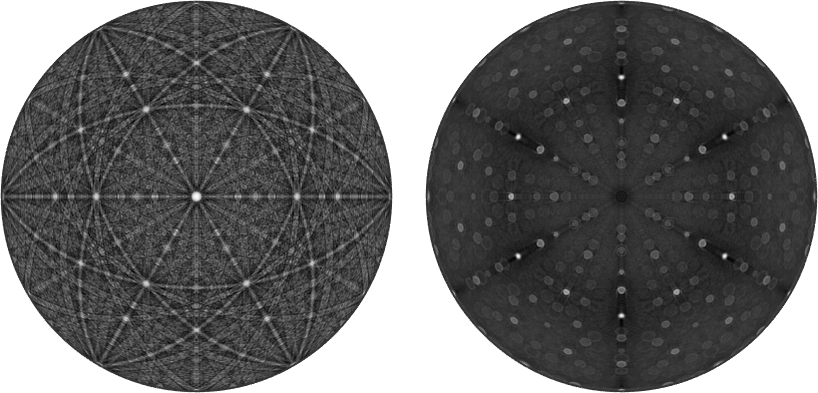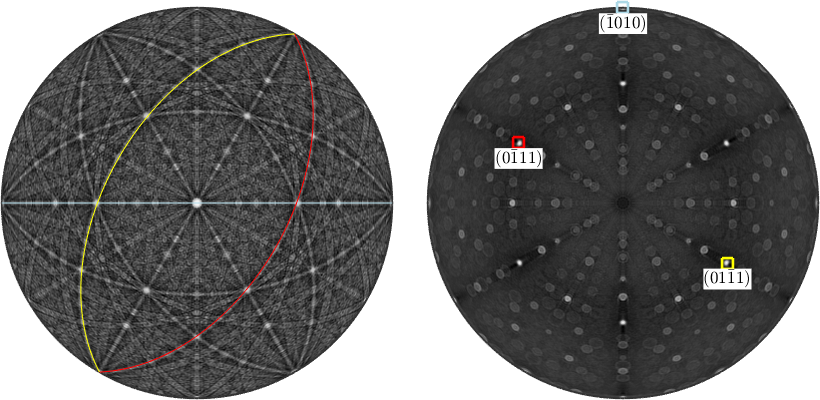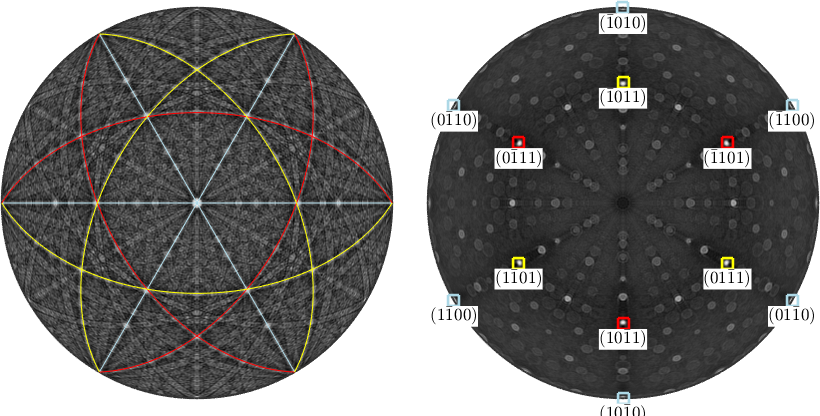Operations Crystal Directions edit page

In this section we discuss basic operations with crystal directions. Therefore, lets start by importing the trigonal Quartz crystal symmetry

and consider two hexgonal prism normal## Zone Axes

Both prism planes intersects in a common zone axis which is orthogonal to both plane normals can is computed by

Note that MTEX automatically switches from reciprocal to direct coordinates for displaying the zone axis.

The other way round two, not paralllel, zone axes

span a lattice plane with normal vector

## Symmetrically Equivalent Crystal Directions

Since crystal lattices are symmetric lattice directions can be grouped into classes of symmetrically equivalent directions. Those groups can be derived by permuting the Miller indeces (uvw). The class of all directions symmetrically equivalent to (uvw) is commonly denoted by uvw, while the class of all lattice planes symmetrically equivalent to the plane (hkl) is denoted by {hkl}. Given a lattice direction or a lattice plane all symmetrically equivalent directions and planes are computed by the command symmetrise

As always the keyword antipodal adds antipodal symmetry to this computation

Using the options symmetrised and labeled all symmetrically equivalent crystal directions are plotted together with their Miller indices. Lets apply this to a list of lattice planesThe command <vector3d.eq.html eq or == > can be used to check whether two crystal directions are symmetrically equivalent. Compare

and

## Angles

The angle between two crystal directions m1 and m2 is defined as the smallest angle between m1 and all symmetrically equivalent directions to m2. This angle is in radians and it is calculated by the function angle

As always the keyword antipodal adds antipodal symmetry to this computation

In order to ignore the crystal symmetry, i.e., to compute the actual angle between two directions use the option noSymmetry

This option is available for many other functions involving crystal directions and crystal orientations.

## Calculations

Essentially all the operations defined for general directions, i.e. for variables of type vector3d are also available for Miller indices. In addition Miller indices interact with crystal orientations. Consider the crystal orientation

Then one can apply it to a crystal direction to find its coordinates with respect to the specimen coordinate system

By applying a crystal symmetry one obtains the coordinates with respect to the specimen coordinate system of all crystallographically equivalent specimen directions.## Conversions

Converting a crystal direction which is represented by its coordinates with respect to the crystal coordinate system a, b, c into a representation with respect to the associated Euclidean coordinate system is done by the command vectord3d.

Conversion into spherical coordinates requires the function polar